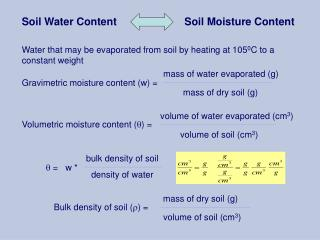DownloadDownload PresentationSoil Water Content

# Soil Water Content

Télécharger la présentation## Soil Water Content

- - - - - - - - - - - - - - - - - - - - - - - - - - - E N D - - - - - - - - - - - - - - - - - - - - - - - - - - -
##### Presentation Transcript

1. Soil Water Content Soil Moisture Content Water that may be evaporated from soil by heating at 1050C to a constant weight mass of water evaporated (g) Gravimetric moisture content (w) = mass of dry soil (g) volume of water evaporated (cm3) Volumetric moisture content (q) = volume of soil (cm3) bulk density of soil q = w * density of water mass of dry soil (g) Bulk density of soil (r) = volume of soil (cm3)

2. Example: A soil is sampled by a cylinder measuring 7.6 cm in diameter and 7.6 cm length. Calculate gravimetric and volumetric water contents, and wet and dry bulk densities using the following data: • Weight of empty cylinder = 300 g • Weight of cylinder + wet soil = 1000 g • Weight of cylinder + oven dry (1050C) soil = 860 g Volume of cylinder = p*r2*h = 3.14*(7.6/2)2*7.6 = 345 cm3 Weight of wet soil = 1000 – 300 = 700 g Weight of dry soil = 860 – 300 = 560 g Dry bulk density = 560/345 = 1.62 g cm-3 Gravimetric moisture content = (700-560)/560 = 0.25 or 25% Volumetric moisture content = r *w = 1.62*0.25 = 0.41 or 41% Know how to do these calculations for quiz on Friday

3. Calculating dry soil weight basis of samples for analysis Weigh drying pan, moist soil subsample + pan, Oven dry the subsample at 105C for 24 hr, Weigh the dried soil + pan. Calculate the moisture content (w): w = (g moist soil – g dry soil)/(g dry soil – pan) Rearrange the eqn to solve for dry soil wt. Dry soil wt = g moist soil / (1 + w)

4. Methods for measuring soil water content Direct method (Gravimetric) Indirect methods (need to calibrate) Electrical properties Acoustic method Thermal properties Chemical methods Radiation technique -Neutron scattering -g- ray attenuation Electrical Conductance Dielectric constant TDR - Gypsum blocks - Nylon blocks - Change in conductance Principles underlying different methods of assessment of soil water content

5. Water Content Direct Gravimetric: evaporating water at 1050C (be able to do the calc’ns) Indirect Neutron scattering: Thermalization Time domain reflectrometry: Dielectric constant

6. Soil Water (matric) Potential In-direct: Watermark (granular matrix sensor), gypsum block Direct: Tensiometer

7. Calibrating field instruments http://www.bae.ncsu.edu/programs/extension/evans/ag452-3.html

8. Gently tap a tube into the soil to take an undisturbed sample from the center of the effective root zone.

9. Trim the soil at each end of the tube to the tube length so that the soil occupies the exact tube volume.

10. Calibration for moisture content • Measure and weigh the tube • Weigh the field moist soil + tube • Oven dry the soil from the tube • Calculate: W = g water/g dry soil = (wet – dry) / dry soil Db = g dry soil / cm3 volume soil Θ = (W x Db) / Dw • Compare lab moisture content to field measurements • For water potential, compare water retention curves derived in lab using pressure plates.

11. Water retention curves: Water content vs pressure or tension Note: clay holds more water at a specific water potential than sand or loam; Water is held tighter at a given water content in clay than in sand. Structure is predominant at low potentials; as soil dries out, texture is more important

12. Effect of structure on water flow www.soils.umn.edu/.../soil2125/doc/s7chp3.htm

13. The flow of water in soil Saturated and unsaturated flow

14. Saturated flow Ksat = Q/A x L/(Ψ1 - Ψ2) where Q is volume of water in time (t) A is area of cross section Ksat is saturated hydraulic conductivity of soil (how fast water moves) L is length of column Ψ is the water potential at points 1 and 2

15. Flux can be thought of as water flowing from a hose. The flux is the rate of water discharged by the hose, divided by the cross-sectional area of the hose. http://soils.usda.gov/technical/technotes/note6fig1.jpg

16. Saturated flow in soils • The pores are full of water and matric potential is considered to be negligible because at least some of the water is a long distance from solid surfaces • Under these conditions, flow is:Rapid - moving through large poresDriven by gravity and sometimesHydrostatic pressure if water is ponded

17. http://www.maf.govt.nz/mafnet/schools/activities/swi/swi-04.htmhttp://www.maf.govt.nz/mafnet/schools/activities/swi/swi-04.htm

18. http://www.montcalm.org/montcalmold/media/planningeduc/tn_gwa5.jpghttp://www.montcalm.org/montcalmold/media/planningeduc/tn_gwa5.jpg

19. Saturation unsaturated wet dry

20. Unsaturated flow Soil moisture content changing with depth

21. Unsaturated flow – most common in soils • Occurs along soil surfaces, not through large pores. • Driven by matric forces that are much stronger than gravity. Gravity is not sufficiently strong to exert a significant influence on unsaturated flow because much of the soil water adheres to solid surfaces. • Unsaturated flow is slow. • Even though the driving force is usually greater than for saturated flow, the resistance to flow is enormous. • Water will flow toward a lower (more negative) potential regardless of direction (up, down, laterally). In other words it will flow towards:            drier medium            salty solution            finer texture (small pores)

22. http://www.maf.govt.nz/mafnet/schools/activities/swi/swi-04.htmhttp://www.maf.govt.nz/mafnet/schools/activities/swi/swi-04.htm

23. http://wwwlb.aub.edu.lb/~webeco/SIM215soilwater_files/image004.gifhttp://wwwlb.aub.edu.lb/~webeco/SIM215soilwater_files/image004.gif